NCERT Maths Solutions for Chapter 3 Ex 3.4 Class 10 acts as the best resource during your learning and helps you score well in your board exams.

## RS Aggarwal Class 10 Solutions Chapter 3 Linear equations in two variables Ex 3D

These Solutions are part of RS Aggarwal Solutions Class 10. Here we have given RS Aggarwal Solutions Class 10 Chapter 3 Linear equations in two variables Ex 3D. You must go through NCERT Solutions for Class 10 Maths to get better score in CBSE Board exams along with RS Aggarwal Class 10 Solutions.

### RS Aggarwal Solutions Class 10 Chapter 3

Show that each of the following systems of equations has a unique solution and solve it:
Question 1.
Solution: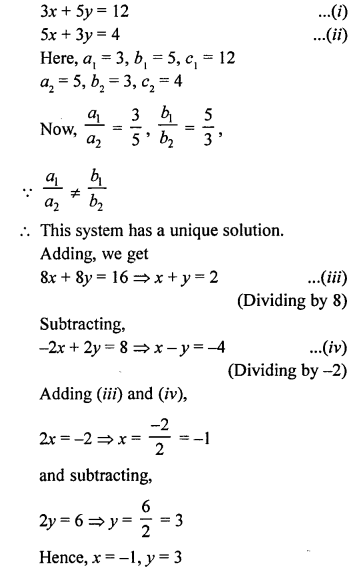Question 2.
Solution: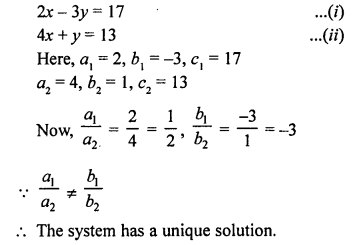Question 3.
Solution: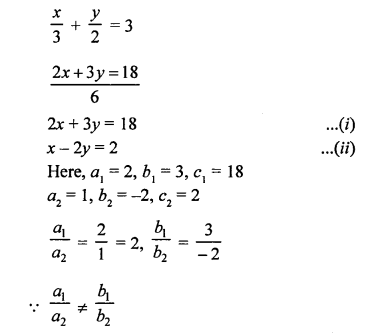This system has a unique solution.
From (ii), x = 2 + 2y
Substituting the value of x in (i),
2(2 + 2y) + 3y = 18
=> 4 + 4y + 3y = 18
=> 7y = 18 – 4 = 14
=> y = 2
and x = 2 + 2 x 2 = 2 + 4 = 6
x = 6, y = 2

Find the value of k for which each of the following systems of equations has a unique solution:
Question 4.
Solution:Question 5.
Solution: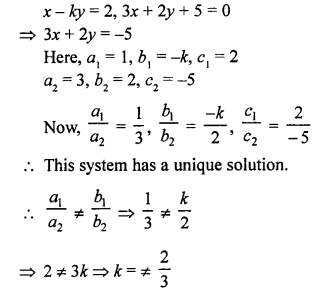Question 6.
Solution: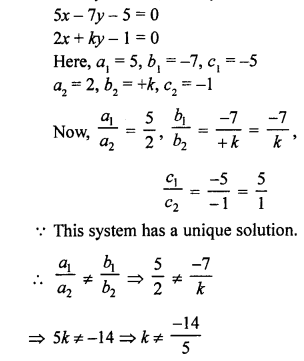Question 7.
Solution: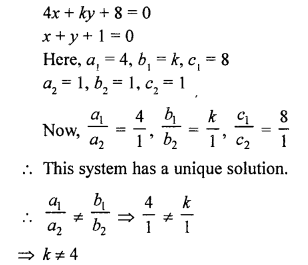Question 8.
Solution: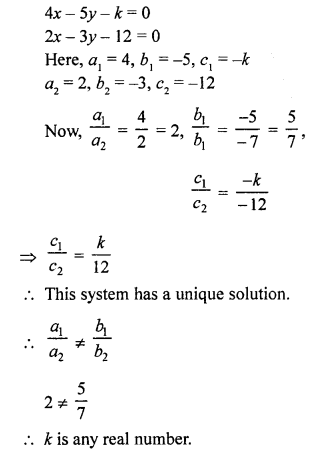Question 9.
Solution: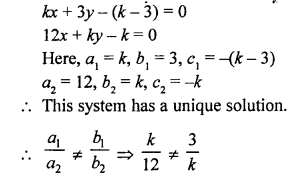Question 10.
Solution: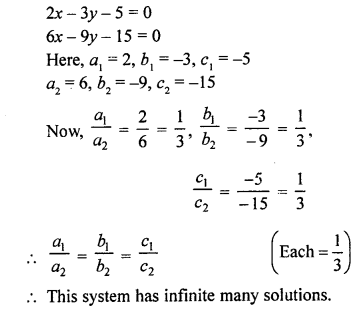Question 11.
Solution: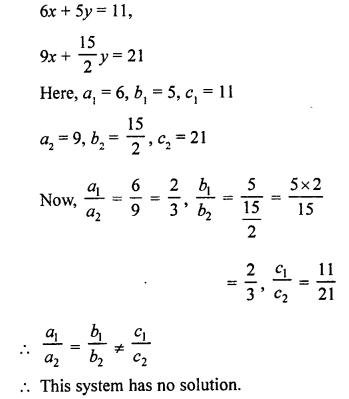Question 12.
Solution: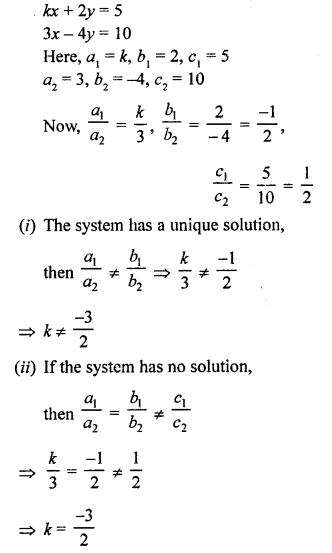Question 13.
Solution: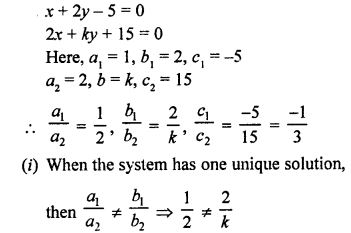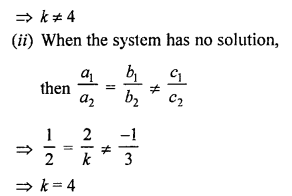Question 14.
Solution: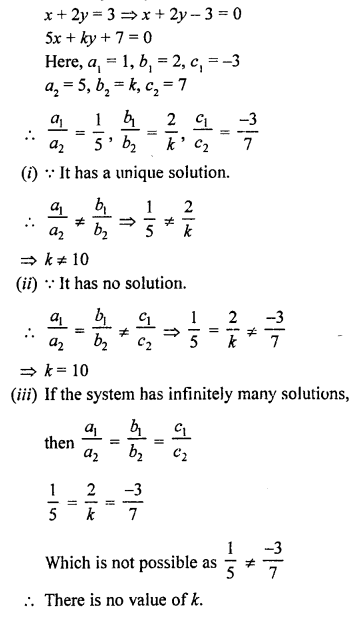Find the value of k for which each of the following systems of linear equations has an infinite number of solutions:
Question 15.
Solution:Question 16.
Solution: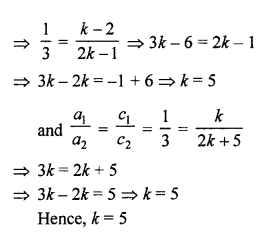Question 17.
Solution: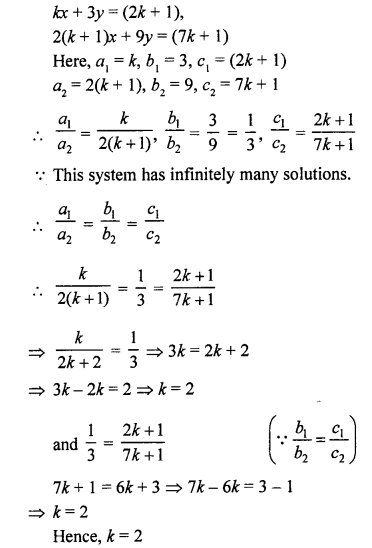Question 18.
Solution: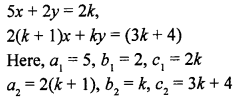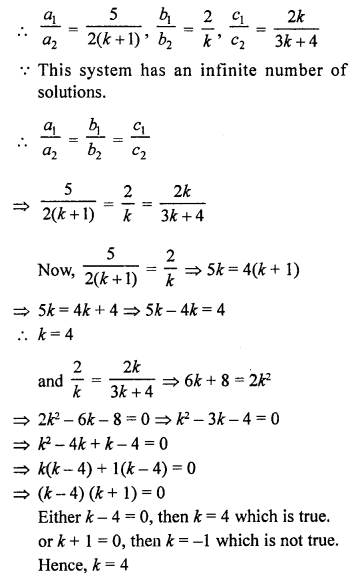Question 19.
Solution: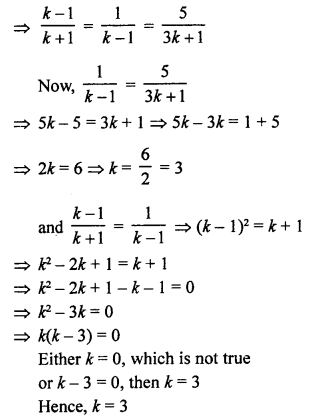Question 20.
Solution:=> k (k – 6) = 0
Either k = 0, which is not true, or k – 6 = 0, then k = 6
k = 6

Find the values of a and b for which each of the following systems of linear equations has an infinite number of solutions:
Question 21.
Solution: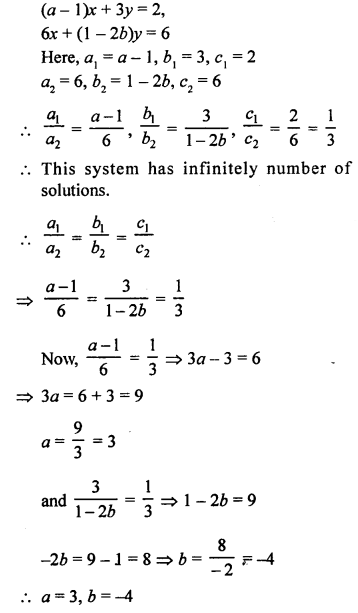Question 22.
Solution: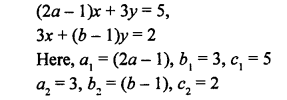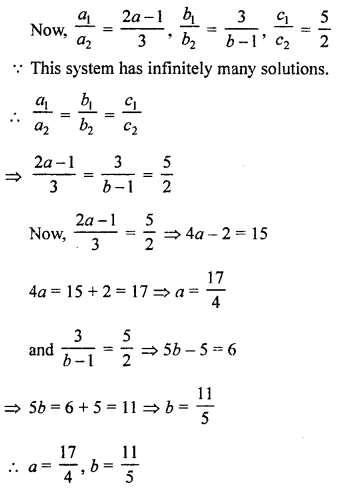Question 23.
Solution:Question 24.
Solution: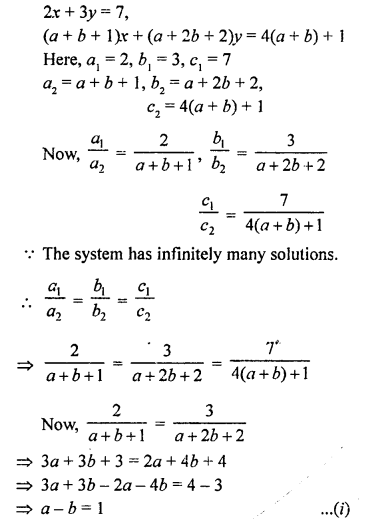Question 25.
Solution:Question 26.
Solution: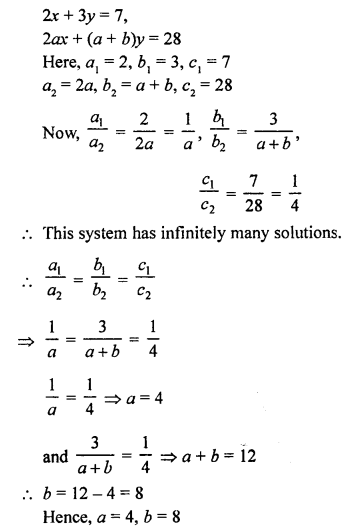Find the value of k for which each of the following systems of equations has no solution:
Question 27.
Solution: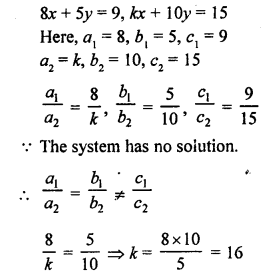Question 28.
Solution:
kx + 3y = 3
12x + ky = 6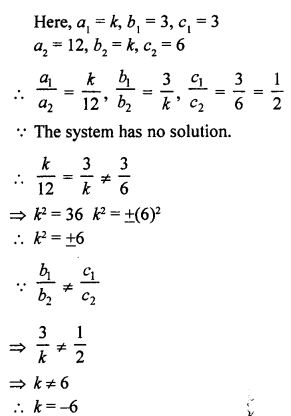Question 29.
Solution: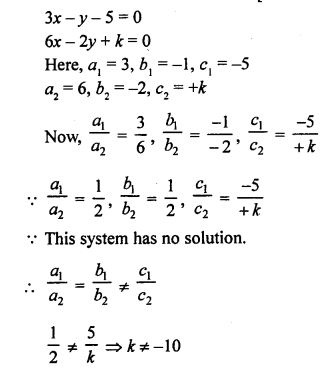Question 30.
Solution: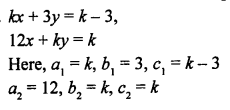Question 31.
Solution: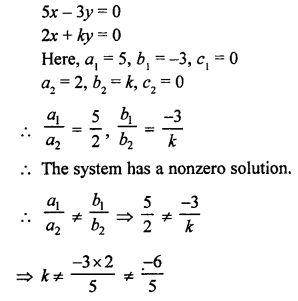Hope given RS Aggarwal Solutions Class 10 Chapter 3 Linear equations in two variables Ex 3D are helpful to complete your math homework.

If you have any doubts, please comment below. Learn Insta try to provide online math tutoring for you.